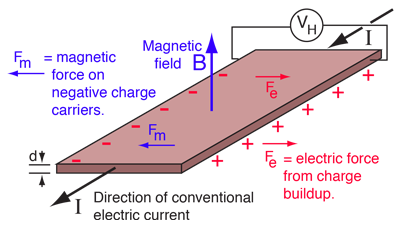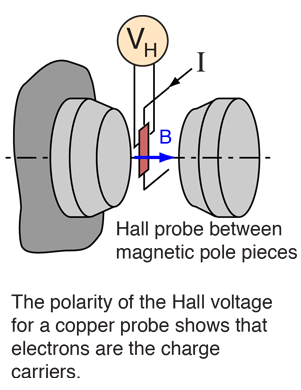# Hall Effect

If an electric current flows through a conductor in a magnetic field, the magnetic field exerts a transverse force on the moving charge carriers which tends to push them to one side of the conductor. This is most evident in a thin flat conductor as illustrated. A buildup of charge at the sides of the conductors will balance this magnetic influence, producing a measurable voltage between the two sides of the conductor. The presence of this measurable transverse voltage is called the Hall effect after E. H. Hall who discovered it in 1879.

Note that the direction of the current I in the diagram is that of conventional current, so that the motion of electrons is in the opposite direction. That further confuses all the "right-hand rule" manipulations you have to go through to get the direction of the forces.The Hall voltage is given by

VH = IB/ned
 Show

where n = density of mobile charges and e = electron charge.

The Hall effect can be used to measure magnetic fields with a Hall probe.

 Hall probe for magnetic field measurement
Index

Magnetic force applications

Magnetic field concepts

 HyperPhysics***** Electricity and Magnetism R Nave
Go Back

# Hall Voltage for Positive Charge Carriers

The transverse voltage (Hall effect) measured in a Hall probe has its origin in the magnetic force on a moving charge carrier.

The magnetic force is Fm = evdB where vd is the drift velocity of the charge.The current expressed in terms of the drift velocity is

I = neAvd
 Show

where n is the density of charge carriers. Then

Fm = eIB/neA

At equilibrium

Fm =Fe=VHe/W
 Show

And substituting gives

VH = IB/ned
Index

Electromagnetic force

Magnetic force

Magnetic field concepts

 HyperPhysics***** Electricity and Magnetism R Nave
Go Back

# Hall Probe

The measurement of large magnetic fields on the order of a Tesla is often done by making use of the Hall effect. A thin film Hall probe is placed in the magnetic field and the transverse voltage (on the order of microvolts) is measured.Sometimes a thin copper film of thickness d on the order of 100 micrometers is used for a Hall probe. Taking the charge carrier density to be

n=8.47x1028 electrons/m3
 Show
For I = amperes
magnetic field B = Tesla
and d = x10^ meters.
The Hall voltage is
VH = IB/ned =
 x10^ volts.
Index

Electromagnetic force

Magnetic force

Magnetic field concepts

 HyperPhysics***** Electricity and Magnetism R Nave
Go Back

# Charge Carriers in the Hall Effect

The Hall effect is a conduction phenomenon which is different for different charge carriers. In most common electrical applications, the conventional current is used partly because it makes no difference whether you consider positive or negative charge to be moving. But the Hall voltage has a different polarity for positive and negative charge carriers, and it has been used to study the details of conduction in semiconductors and other materials which show a combination of negative and positive charge carriers.

The Hall effect can be used to measure the average drift velocity of the charge carriers by mechanically moving the Hall probe at different speeds until the Hall voltage disappears, showing that the charge carriers are now not moving with respect to the magnetic field. Other types of investigations of carrier behavior are studied in the quantum Hall effect.

Index

Electromagnetic force

Magnetic force

Magnetic field concepts

 HyperPhysics***** Electricity and Magnetism R Nave
Go Back